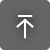# TL Hilbert Curve Generator

Todor Latev

OS:

## 説明

From Wikipedia:

A Hilbert curve (also known as a Hilbert space-filling curve) is a continuous fractal space-filling curve first described by the German mathematician David Hilbert in 1891.  It is a variant of the space-filling Peano curves discovered by Giuseppe Peano in 1890.

Because it is space-filling, its Hausdorff dimension is {\displaystyle 2}. Precisely described, its image is the unit square, whose dimension is 2 in any definition of dimension; its graph is a compact set homeomorphic to the closed unit interval, with Hausdorff dimension 2).

This app recursively generates both 2D and 3D Hilbert space-filling fractal pseudo curves. The curve level is specified by the user and a delay factor can also be supplied which results in an animation like curve generation. The scale factor along the X and Y need not be the same.

The resulting pattern can be used as a hatch pattern in Autodesk® AutoCAD®.

This specific space-filling curve has some interesting and useful properties with a wide range of applications

To learn more visit: https://en.wikipedia.org/wiki/Hilbert_curve

ヘルプ ドキュメントを読む

## このバージョンについて

バージョン 2018.6.25, 2021/05/06
Added 2022 support

## カスタマ レビュー

(0 件のレビュー)

### |

テクニカル ヘルプを表示